# Uncertainty Principle and Vacuum Polarization

The uncertainty in energy can be as large as you like as long as the time interval is very short: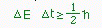If the time interval is short enough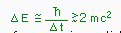then the force carrying particle can transmute itself into another particle-antiparticle pair.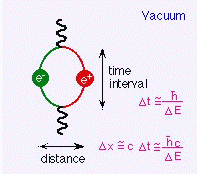As you go up in energy, you will be able to see phenomena that happen at shorter distance and in shorter time interval.

# Coupling Constant and Energy (In the Case of Electromagnetic Force)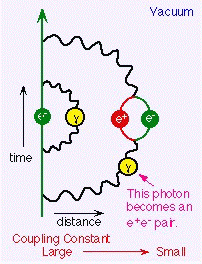Force carrying particles turn into other particle-antiparticle pairs momentarily. Then the number of force carrying particles becomes smaller and the force becomes weaker.

The photons emitted from the electron at times transmute themselves to e+e- pairs momentarily. The probability of this happening is larger as the flight length is longer. The number of force carrying particles thus depends on the distance or equivalently the energy scale.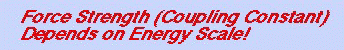# Coupling Constant and Energy (In the Case of Strong Force)

As with the electromagnetic interaction, the quark-antiquark pairs created via vacuum polarization reduce the number of force carrying particles and thus make the coupling constant smaller at a larger distance. But the force carriers of the strong force (gluons) have color charges themselves, unlike the photon mediating the electromagnetic force, and are able to juggle other gluons and increase the number of force carrying particles.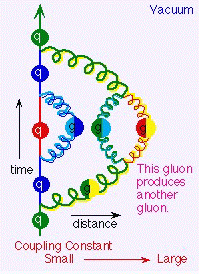The effect of gluons' self-duplications dominates the effect of quark-antiquark pairs' reducing the number of gluons and the number of force carrying particles gets larger with distance. Thus the coupling constant becomes larger at lower energies and it becomes infinitely strong eventually (color confinement).

# Unification of Forces

When we extrapolate the recent measurements of the coupling constants to the energy of the beginning of the universe, assuming supersymmetry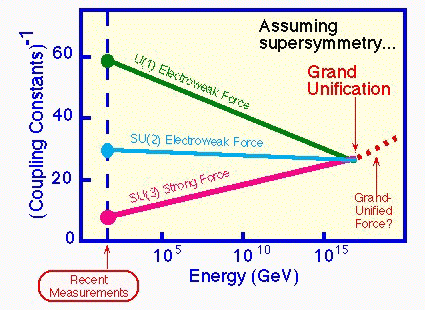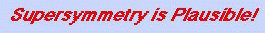webmaster@www-jlc.kek.jp Feb 08, 1995